# ActiveShell: Solving a Small Programming Exercise

(This is a followup to Introducing ActiveShell.)

ActiveShell is still in the prototype stage, and everything described in this post is likely to change. Most of the I/O ports to read and write data from elsewhere haven't been written yet. However, even without pulling much data from the Web, we can solve programming puzzles. This one is from the excellent Stanford online ai-class.

In this puzzle, we are given a secret message which has been encoded with a rotation (or Caesar or shift) cypher, of which rot13 is an example. The full problem description as given by Peter Norvig can be heard on the ai-class site. I'll solve only the first of the two parts.

The only input we need is the secret message: "Esp qtcde nzyqpcpynp zy esp ezatn zq Lcetqtntlw Tyepwwtrpynp hld spwo le Olcexzfes Nzwwprp ty estd jplc."

Let's begin with an empty shell session opened in the browser: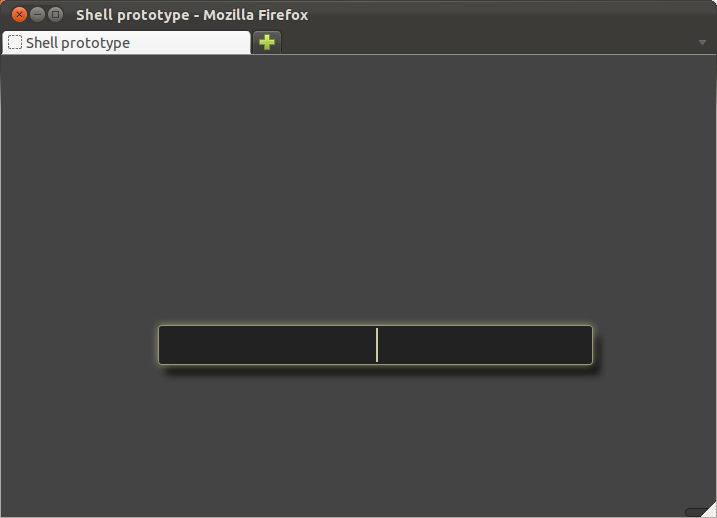First, a note about syntax. Unix shells have complex grammars that I think most of us who use them would be glad to forget. Modern programming language syntaxes tends to be more consistent, but also more complex than what a shell requires. Given that ActiveShell is aiming to improve the state of the art in shells, I think it's worth using a new syntax. However, only the parsed representation is stored, so we can freely experiment or even allow per-user syntax in the future.

## The ActiveShell Grammar

A command consists of (in any order) one verb and zero or more arguments. The order of the arguments relative to each other does matter, but the verb can appear before, during, or after the arguments. An argument is either a literal or a reference. A literal is always in double quotes, with any double quote in the value itself preceded by a backslash. A reference is a bare number, as we will see below. A verb is a sequence of lower case letters 'a' to 'z'. Capital 'A' through 'Z' are used for ports, which I'm ignoring in this post.

## End Grammar

That is the whole ActiveShell command syntax in one paragraph. It is simple and small, but the use of references gives it power. Commands don't contain other commands, and one command with exactly one verb is given at a time. That means there is no pipe syntax, no command substitution, much less shell syntax to learn, and fewer edge cases to remember. Pipe syntax is rendered unnecessary by a more general feature whereby commands can refer to previous commands. However, all characters not mentioned above are reserved, so there is plenty of room to add more syntax later. Before doing so, I want to see how far the minimal syntax with references can go.

Now, on to the task. We need to load some verbs, which is something like installing binaries in Unix. I'll also paste the secret message into the shell: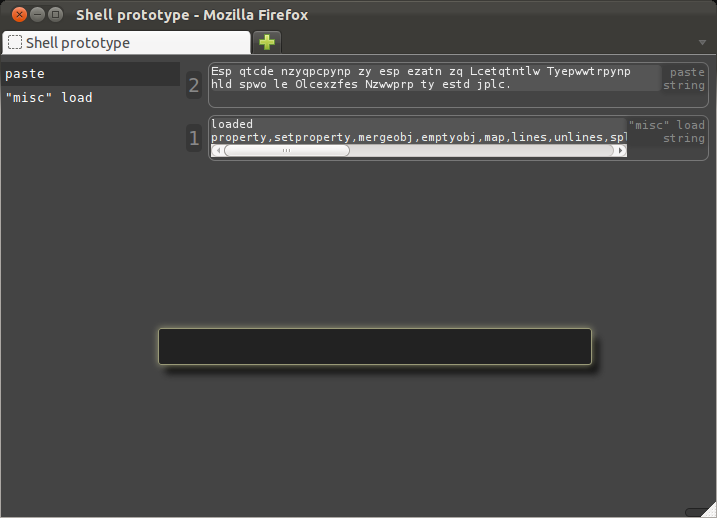In case you are wondering how verbs are implemented, the verbs we just loaded are stored as JSON, which the shell just fetched and parsed. Here's the JSON for "split":

``````
...
"split":
{"desc":"split a string by a delimiter"
,"args":["input:String","delimiter:String"]
,"outs":["substrings:[String]"]
,"f":"function(cmd){cmd.out(cmd.args.split(cmd.args))}"
}
...
``````

Now we are ready to play with the data and see if we can solve the problem.

There are 26 letters in the Latin alphabet, so there are 26 possible rotations, including the 0 rotation, and we know one of them will be the answer.

Here we generate the list of possibilities. Note that "26" is quoted, because it is a literal argument, not a reference: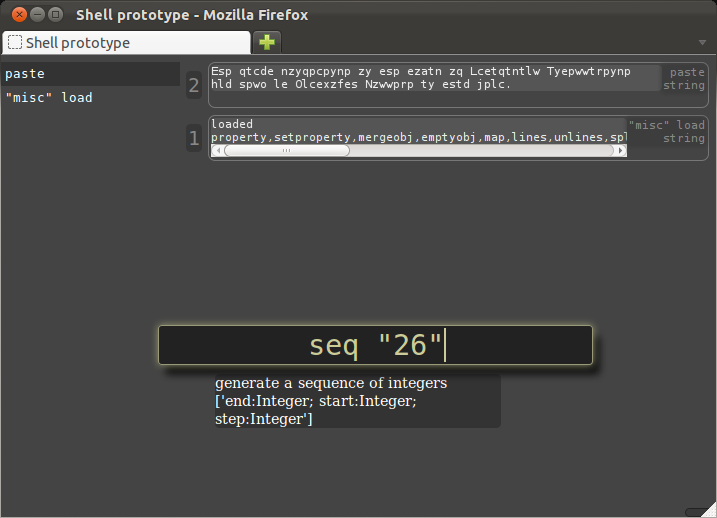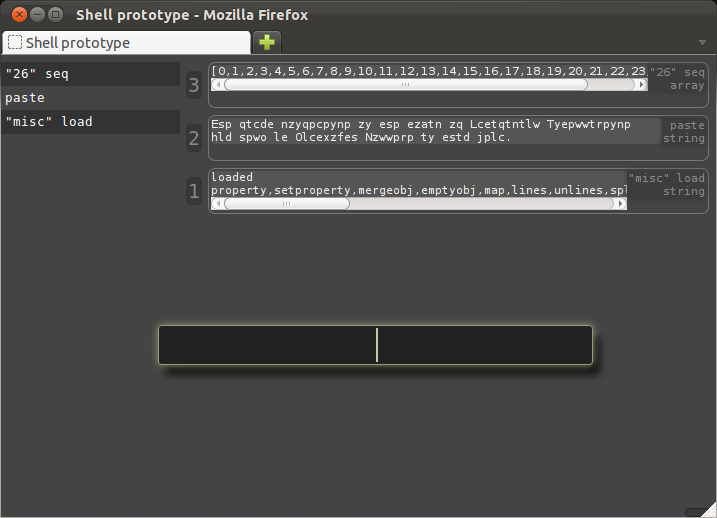Now let's take an element from that sequence to test. Since rotating by 0 isn't very exciting, I'll take the last element. Here the bare number 3 is not quoted, because is a reference to the previous command rather than a literal argument.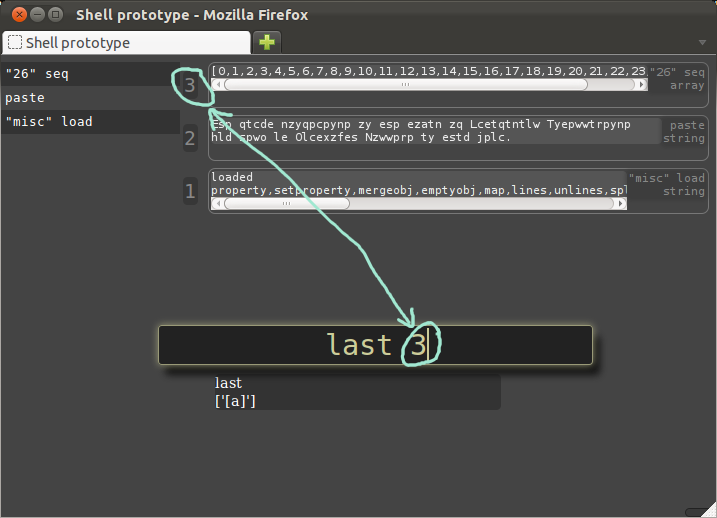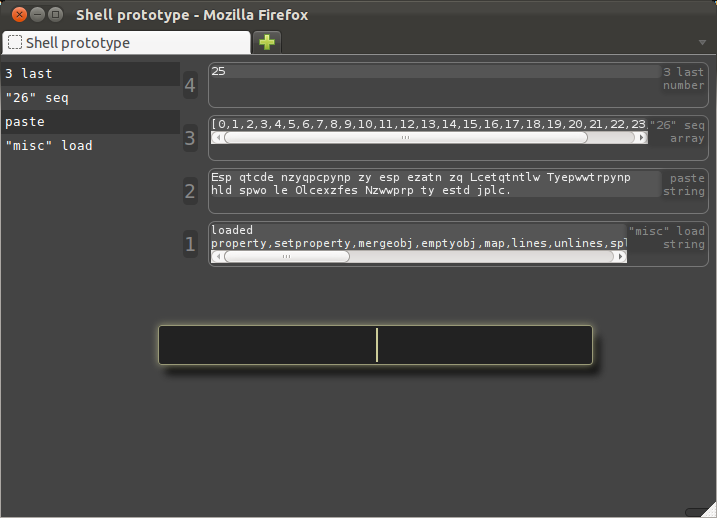Now we can test the rotation verb that I just wrote for this demo, and see if it actually works. It takes two arguments: the amount to rotate, and the input string. Both of these arguments will again be references from previous commands: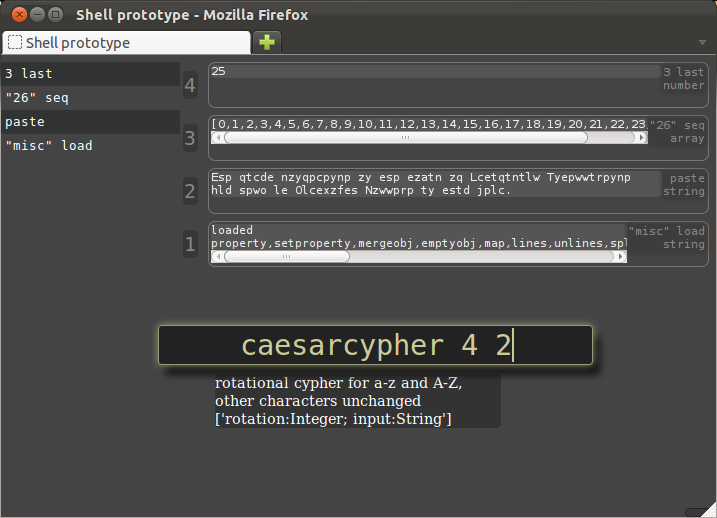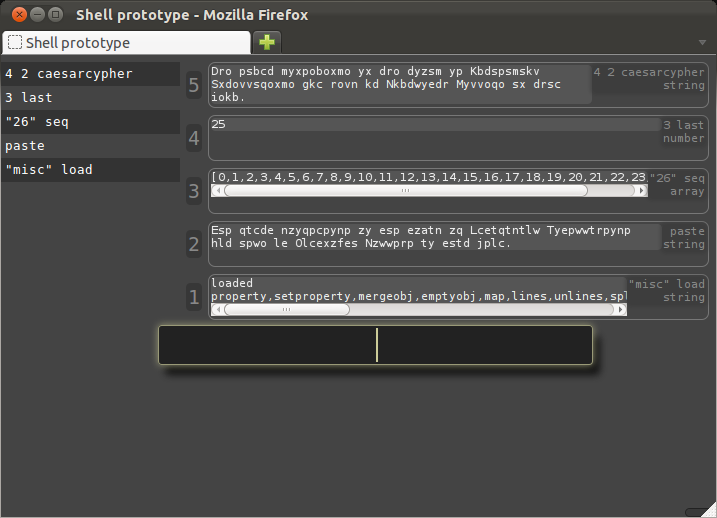It seems to work, correctly changing the first letter from an 'E' to a 'D', which is one earlier in the alphabet, or 25 later after wrapping around.

Now, we want to do the same thing again, but for each of the 26 possibilities instead of just the last possibility.

Here is where we might expect to need a loop, but instead we can use references to previous commands, specifically the last three: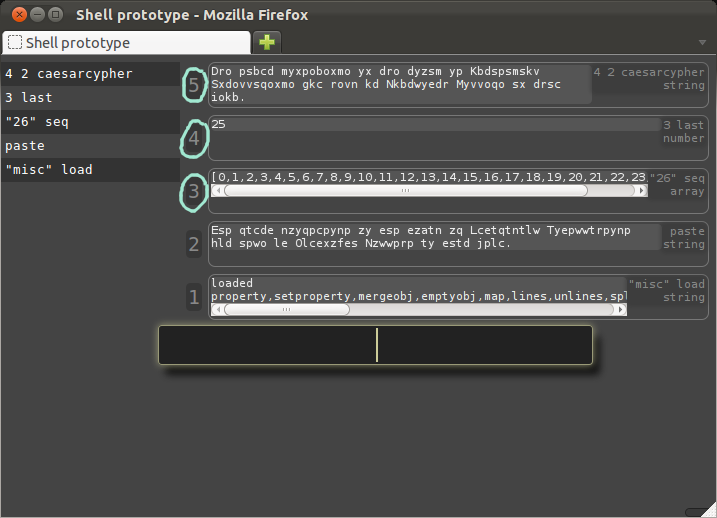If we were giving instruction to another person, we would say something like "now do that same thing for each of these". To give that same instruction to a shell, we need a way to specify "that same thing" and "each of these", both of which we can do by directly referencing previous commands, in this case 3, 4, and 5.

Specifically, the result we want here is something like 5, but where previously we used only 4 as an input, we now want to use each of the elements of 3 as the input. We can express that with the "each" verb as "each 5 4 3":(The hinting of what is about to be done can certainly be far improved from what the prototype shows above.)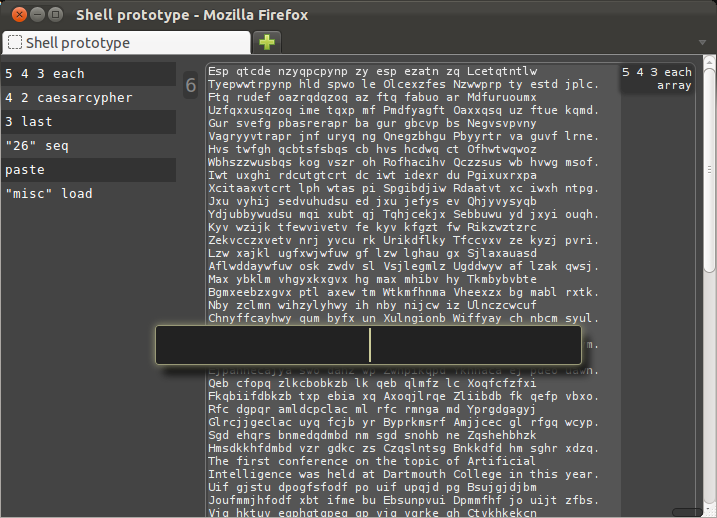This example-based approach to looping has some advantages, not the least of which is that getting the result we want often takes more steps than expected. Rather than rewrite a loop until it works, here we loop only after making the loop body work correctly (for at least one input value). This example-based programming style works well for exploration and typical ad-hoc shell programming tasks.

Now that we have our output, we could just scan the results visually and find the one that is in English. In the spirit of the question, though, let's pretend we can't, and use a more sophisticated technique instead.

First we want to generate a language model which we can use to score these 26 result strings as more or less English-like. I'll be using character 4-grams as the model. I've written two verbs: ngrams and ngramlikelihood. The details are not important here, but the first verb takes an input string and generates a statistical model from it. The second takes that statistical model and another string and gives a score indicating how likely that string is under that statistical model. This gives us a very rough way to measure how likely a string is to be English, which while fairly weak, is probably good enough to distinguish English from rotated gibberish.

Before we can generate a statistical model of English, we need some English text to generate our n-gram model from. For this I'll use the text of Wikipedia article on Natural Language Processing.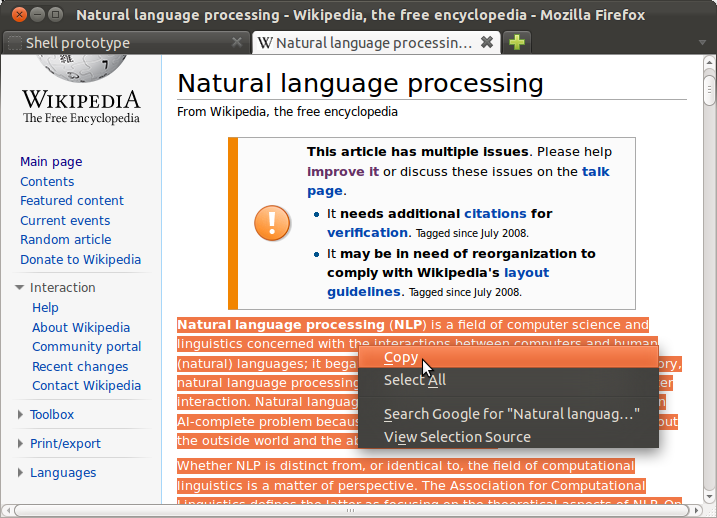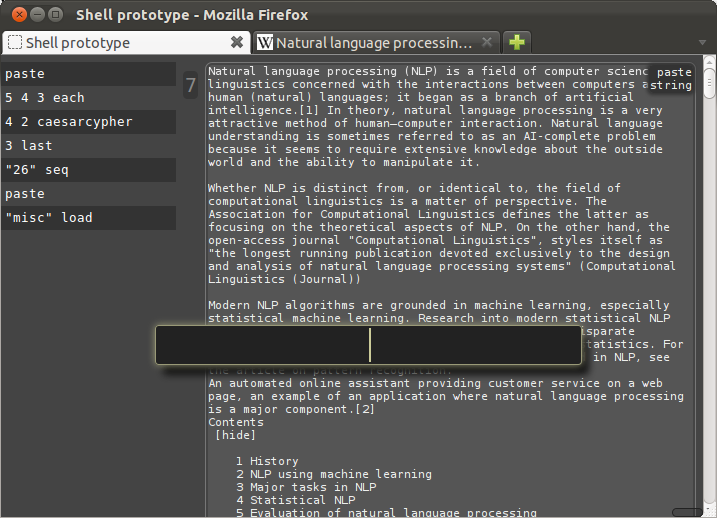Now that we have our representative English sample, we can count the 4-grams that occur in it, and then use this model to score our original input string with the ngramlikelihood verb: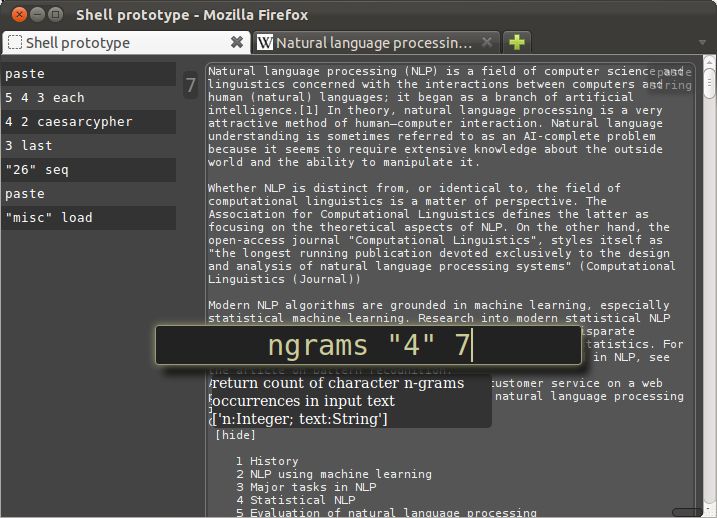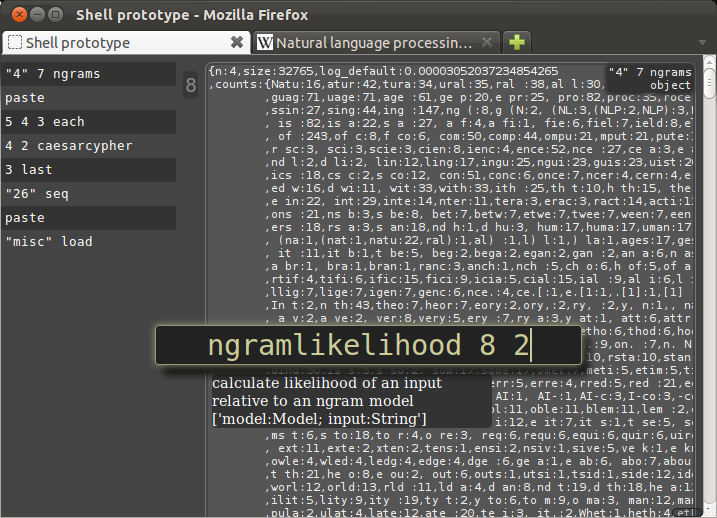The output of ngramlikelihood, 3.69, is an unscaled value, and doesn't mean much except in relation to the scores of the other strings.

To score the rest of the strings, we could use "each" again, but I'm going to use a single command which both iterates over and also sorts the values, with the sortby verb. Like "each", "sortby" takes three arguments, which are the list to be sorted, the score that was calculated, and the input that the score was calculated from. In this case, the list is in 6, the score we are sorting by was calculated in the previous command, 9, and it was calculated using 2, the original string, as an input. The command below could be read as "Each element in six, sort by nine as calculated from two." The rotation with the highest score should be the string we want.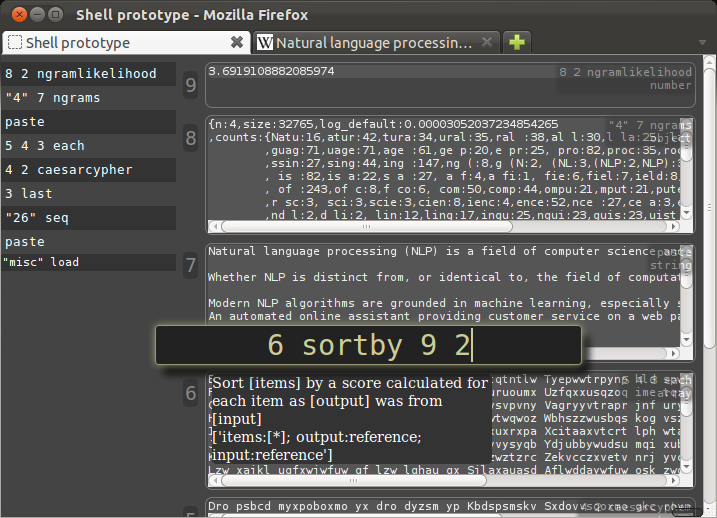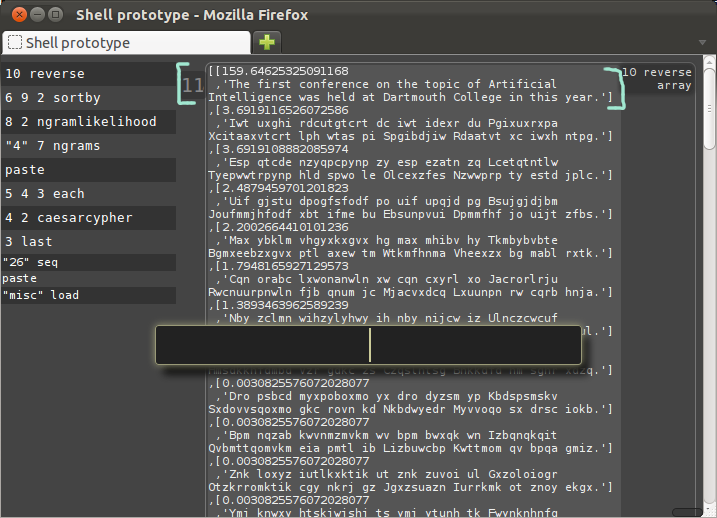And there at the top is the decoded message. Even this very simple language model gave quite a difference in score between the correct answer at 159.6 and the next best at 3.7.

Now that we've decoded the secret message, this demo is done. The most significant feature we didn't cover here is ports, which allow you to pull data into the shell from the network or from the local machine (in fact the 'paste' and 'load' verbs used above require the browser port).

Future demos will cover ports as they become available.